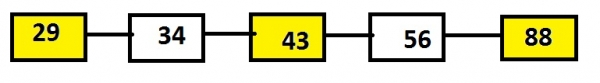# Print the alternate nodes of linked list (Iterative Method) in C language

In this problem, program must print the alternates from the given linked list that is leaving one printing other and so on using iterative method.

Iterative method is the one which generally uses loops that are executed till the condition holds value 1 or true.

Let’s say, list contains the nodes 29, 34, 43, 56 and 88 and than the output will be the alternate nodes such as 29, 43 and 88.## Example

Input: 29->34->43->56->88
Output: 29 43 88

The approach is to traverse the entire list till the last node. While, traversing a counter variable can be taken which is incremented to 1 and value is printed when the counter is even or odd depending upon user’s choice. If the user wants it to display from the 0 than the counter with even value is displayed else the counter with odd value is displayed.

The below code shows the c implementation of the algorithm given.

## Algorithm

START
Step 1 -> create node variable of type structure
Declare int data
Declare pointer of type node using *next
Step 2 -> Declare Function void alternate(struct node* head)
Set int count = 0
IF count % 2 = 0
Set count++
End
Step 3 -> Declare Function void push(struct node** header, int newdata)
Create newnode using malloc function
Set newnode->data = newdata
step 4 -> In Main()
create head pointing to first node using struct node* head = NULL
STOP

## Example

#include <stdio.h>
#include <stdlib.h>
//creating structure of a node
struct node {
int data;
struct node* next;
};
//function to find and print alternate node
int count = 0;
if (count % 2 == 0)
count++;
}
}
//pushing element into the list
void push(struct node** header, int newdata) {
struct node* newnode =
(struct node*)malloc(sizeof(struct node));
newnode->data = newdata;
}
int main() {
printf("alternate nodes are :");
push(&head, 1); //calling push function to push elements in list
}
alternate nodes are : 80 10 1